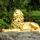## Social Question# What do you think of this Singapore math problem?

Asked by LostInParadise (28821) 1 month ago

Students from Singapore get the highest scores in international math tests. The claim is that this is largely due to a technique that they use before teaching algebra known as block modeling. I have been looking at several examples online. The problems are easy to solve algebraically, but I am having a hard time using block modeling.

Here is an example of a 7th grade math problem. 3/5 of the men at a conference are from Georgia and ⅓ of the women are from Georgia. The number of men from Georgia is the same as the number of women from Georgia and there are 8 more women than men. How many people are from Georgia?

You might want to pause for a moment and work this out on your own. Algebraically, I let x = number of men. Then we get:
3/5 x = ⅓(x+8).
x works out to be 10. There are 3/5x=6 men from Georgia and an equal number of women for a total of 12.

Here is the diagram used for the Singapore approach. The top row shows 3 of the 5 men boxes representing the 3/5 of the men from Georgia. Since the number of women from Georgia is the same as the number of men from there, 3 boxes also represent the number of women from Georgia.

Because ⅓ of the women are from Georgia, we use 9 boxes to represent the total number of women. The women have 4 more boxes than the men and since there are 8 more women than men, each of those boxes must stand for 2 people. There are 6 boxes representing people from Georgia, so there must be a total of 6×2=12 people from there.

Observing members: 0Composing members: 0[ Insert joke here about Singapore government denying existence of non-conformist gender identity here]

I was almost always very good at math, and my approach was usually to try to relate to the problem as an actual thing. Sketching a problem can be quite helpful, as it can give you a handle on it that numbers and equations won’t, at least for some people. So I expect yes, that “Signapore block” system is probably very helpful, though the diagram bothers me a little since it uses one block for every two people.

Zaku (26628)“Great Answer” (2) Flag as…